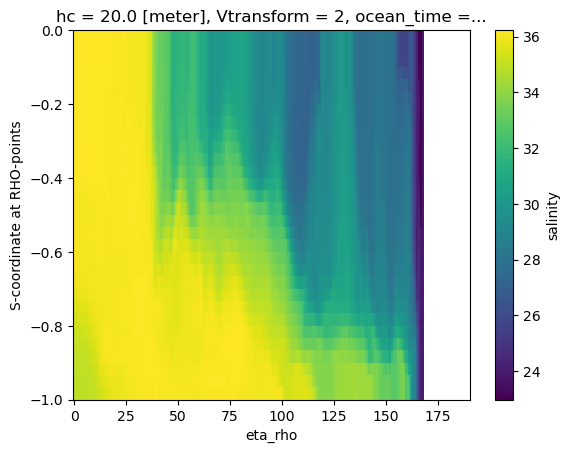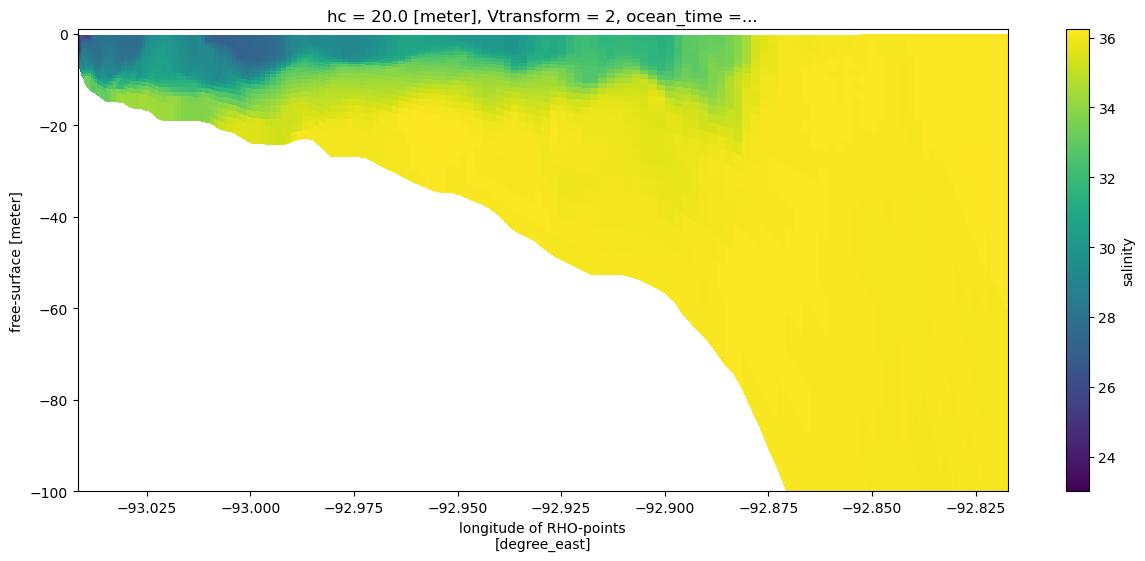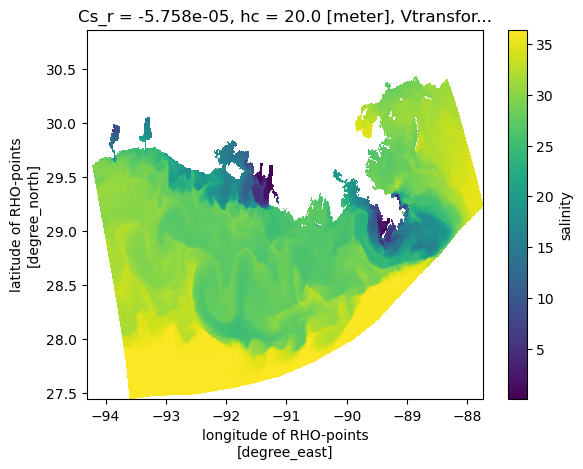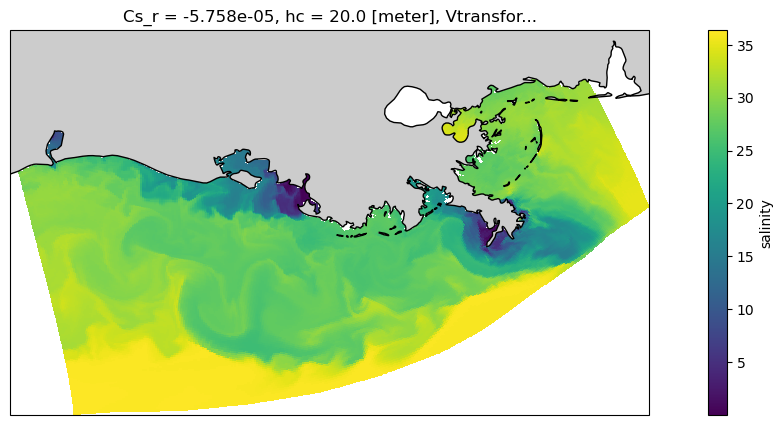You can run this notebook in a live sessionor view it on Github.

# ROMS Ocean Model Example#

The Regional Ocean Modeling System (ROMS) is an open source hydrodynamic model that is used for simulating currents and water properties in coastal and estuarine regions. ROMS is one of a few standard ocean models, and it has an active user community.

ROMS uses a regular C-Grid in the horizontal, similar to other structured grid ocean and atmospheric models, and a stretched vertical coordinate (see the ROMS documentation for more details). Both of these require special treatment when using xarray to analyze ROMS ocean model output. This example notebook shows how to create a lazily evaluated vertical coordinate, and make some basic plots. The xgcm package is required to do analysis that is aware of the horizontal C-Grid.

:

import numpy as np
import cartopy.crs as ccrs
import cartopy.feature as cfeature
import matplotlib.pyplot as plt

%matplotlib inline

import xarray as xr


Load a sample ROMS file. This is a subset of a full model available at

http://barataria.tamu.edu/thredds/catalog.html?dataset=txla_hindcast_agg


The subsetting was done using the following command on one of the output files:

#open dataset
ds = xr.open_dataset('/d2/shared/TXLA_ROMS/output_20yr_obc/2001/ocean_his_0015.nc')

# Turn on chunking to activate dask and parallelize read/write.
ds = ds.chunk({'ocean_time': 1})

# Pick out some of the variables that will be included as coordinates
ds = ds.set_coords(['Cs_r', 'Cs_w', 'hc', 'h', 'Vtransform'])

# Select a a subset of variables. Salt will be visualized, zeta is used to
# calculate the vertical coordinate
variables = ['salt', 'zeta']
ds[variables].isel(ocean_time=slice(47, None, 7*24),
xi_rho=slice(300, None)).to_netcdf('ROMS_example.nc', mode='w')


So, the ROMS_example.nc file contains a subset of the grid, one 3D variable, and two time steps.

## Load in ROMS dataset as an xarray object#

:

# load in the file
ds = xr.tutorial.open_dataset("ROMS_example.nc", chunks={"ocean_time": 1})

# This is a way to turn on chunking and lazy evaluation. Opening with mfdataset, or
# setting the chunking in the open_dataset would also achieve this.
ds

:

<xarray.Dataset>
Dimensions:     (ocean_time: 2, s_rho: 30, eta_rho: 191, xi_rho: 371)
Coordinates:
Cs_r        (s_rho) float64 dask.array<chunksize=(30,), meta=np.ndarray>
lon_rho     (eta_rho, xi_rho) float64 dask.array<chunksize=(191, 371), meta=np.ndarray>
hc          float64 ...
h           (eta_rho, xi_rho) float64 dask.array<chunksize=(191, 371), meta=np.ndarray>
lat_rho     (eta_rho, xi_rho) float64 dask.array<chunksize=(191, 371), meta=np.ndarray>
Vtransform  int32 ...
* ocean_time  (ocean_time) datetime64[ns] 2001-08-01 2001-08-08
* s_rho       (s_rho) float64 -0.9833 -0.95 -0.9167 ... -0.05 -0.01667
Dimensions without coordinates: eta_rho, xi_rho
Data variables:
salt        (ocean_time, s_rho, eta_rho, xi_rho) float32 dask.array<chunksize=(1, 30, 191, 371), meta=np.ndarray>
zeta        (ocean_time, eta_rho, xi_rho) float32 dask.array<chunksize=(1, 191, 371), meta=np.ndarray>
Attributes: (12/34)
file:              ../output_20yr_obc/2001/ocean_his_0015.nc
format:            netCDF-4/HDF5 file
Conventions:       CF-1.4
type:              ROMS/TOMS history file
title:             TXLA ROMS hindcast run with dyes and oxygen
rst_file:          ../output_20yr_obc/2001/ocean_rst.nc
...                ...
compiler_flags:    -heap-arrays -fp-model fast -mt_mpi -ip -O3 -msse2 -free
tiling:            010x012
history:           Tue Jul 24 11:04:43 2018: /opt/nco/ncks -D 4 -t 8 /cop...
ana_file:          /home/d.kobashi/TXLA_ROMS_reana/Functionals/ana_btflux...
CPP_options:       TXLA2, ANA_BPFLUX, ANA_BSFLUX, ANA_BTFLUX, ANA_NUDGCOE...
NCO:               netCDF Operators version 4.7.6-alpha04 (Homepage = htt...

## Add a lazilly calculated vertical coordinates#

Write equations to calculate the vertical coordinate. These will be only evaluated when data is requested. Information about the ROMS vertical coordinate can be found (here)[https://www.myroms.org/wiki/Vertical_S-coordinate]

In short, for Vtransform==2 as used in this example,

$$Z_0 = (h_c \, S + h \,C) / (h_c + h)$$

$$z = Z_0 (\zeta + h) + \zeta$$

where the variables are defined as in the link above.

:

if ds.Vtransform == 1:
Zo_rho = ds.hc * (ds.s_rho - ds.Cs_r) + ds.Cs_r * ds.h
z_rho = Zo_rho + ds.zeta * (1 + Zo_rho / ds.h)
elif ds.Vtransform == 2:
Zo_rho = (ds.hc * ds.s_rho + ds.Cs_r * ds.h) / (ds.hc + ds.h)
z_rho = ds.zeta + (ds.zeta + ds.h) * Zo_rho

ds.coords["z_rho"] = z_rho.transpose()  # needing transpose seems to be an xarray bug
ds.salt

:

<xarray.DataArray 'salt' (ocean_time: 2, s_rho: 30, eta_rho: 191, xi_rho: 371)>
dask.array<open_dataset-d19147b4d8cb936f6c04a1c63ed2764asalt, shape=(2, 30, 191, 371), dtype=float32, chunksize=(1, 30, 191, 371), chunktype=numpy.ndarray>
Coordinates:
Cs_r        (s_rho) float64 dask.array<chunksize=(30,), meta=np.ndarray>
lon_rho     (xi_rho, eta_rho) float64 dask.array<chunksize=(371, 191), meta=np.ndarray>
hc          float64 20.0
h           (xi_rho, eta_rho) float64 dask.array<chunksize=(371, 191), meta=np.ndarray>
lat_rho     (xi_rho, eta_rho) float64 dask.array<chunksize=(371, 191), meta=np.ndarray>
Vtransform  int32 2
* ocean_time  (ocean_time) datetime64[ns] 2001-08-01 2001-08-08
* s_rho       (s_rho) float64 -0.9833 -0.95 -0.9167 ... -0.05 -0.01667
z_rho       (s_rho, xi_rho, eta_rho, ocean_time) float64 dask.array<chunksize=(30, 371, 191, 1), meta=np.ndarray>
Dimensions without coordinates: eta_rho, xi_rho
Attributes:
long_name:  salinity
time:       ocean_time
field:      salinity, scalar, series

## A naive vertical slice#

Creating a slice using the s-coordinate as the vertical dimension is typically not very informative.

:

ds.salt.isel(xi_rho=50, ocean_time=0).plot()

:

<matplotlib.collections.QuadMesh at 0x7fbd485c6bb0>We can feed coordinate information to the plot method to give a more informative cross-section that uses the depths. Note that we did not need to slice the depth or longitude information separately, this was done automatically as the variable was sliced.

:

section = ds.salt.isel(xi_rho=50, eta_rho=slice(0, 167), ocean_time=0)
section.plot(x="lon_rho", y="z_rho", figsize=(15, 6), clim=(25, 35))
plt.ylim([-100, 1]);## A plan view#

Now make a naive plan view, without any projection information, just using lon/lat as x/y. This looks OK, but will appear compressed because lon and lat do not have an aspect constrained by the projection.

:

ds.salt.isel(s_rho=-1, ocean_time=0).plot(x="lon_rho", y="lat_rho")

:

<matplotlib.collections.QuadMesh at 0x7fbd4833c940>And let’s use a projection to make it nicer, and add a coast.

:

proj = ccrs.LambertConformal(central_longitude=-92, central_latitude=29)
fig = plt.figure(figsize=(15, 5))
ax = plt.axes(projection=proj)
ds.salt.isel(s_rho=-1, ocean_time=0).plot(
x="lon_rho", y="lat_rho", transform=ccrs.PlateCarree()
)

coast_10m = cfeature.NaturalEarthFeature(
"physical", "land", "10m", edgecolor="k", facecolor="0.8"
)

:

<cartopy.mpl.feature_artist.FeatureArtist at 0x7fbd4826a8e0>

/home/docs/checkouts/readthedocs.org/user_builds/xray/conda/stable/lib/python3.9/site-packages/cartopy/io/__init__.py:241: DownloadWarning: Downloading: https://naturalearth.s3.amazonaws.com/10m_physical/ne_10m_land.zip[ ]: